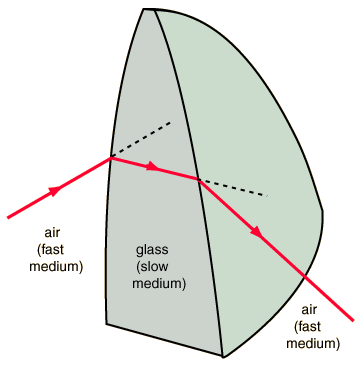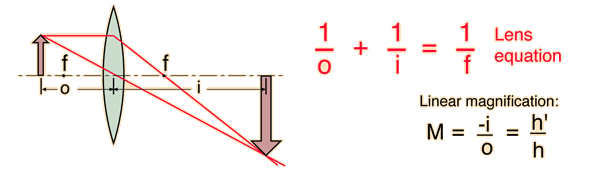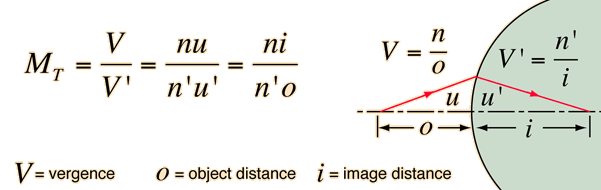# Refraction by a Convex Lens

Refraction bends the light downward upon entering the glass because the bottom part of the ray hits the slow medium first.

Light travels more slowly in glass than in air. The amount of bending depends upon the index of refraction of the glass.Image formation depends upon bending light rays with lenses.

Refraction bends the light downward again upon leaving the glass because the top part of the ray hits the faster medium first.

Index

Lens concepts

 HyperPhysics***** Light and Vision R Nave
Go Back

# Magnification:Transverse &Angular

The linear magnification or transverse magnification is the ratio of the image size to the object size. If the image and object are in the same medium it is just the image distance divided by the object distance.Using the Gaussian form of the lens equation, a negative sign is used on the linear magnification equation as a reminder that all real images are inverted. If the image is virtual, the image distance will be negative, and the magnification will therefore be positive for the erect image.

If the media are different on the two sides of the surface or lens, the magnification is not quite so straigtforward. It can be variously expressed asIn this equation V is the vergence, n is the index of refraction, and u is used for the angle. Note that in this expression for the magnification, the minus sign doesn't appear. In this type of setting, the Cartesian sign convention is typically used and the object distance has a negative value. That takes care of the minus sign that is put in above in the Gaussian form.

The angular magnification of an instrument is the ratio of the angle subtended at the eye when using the instrument divided by the angular size without the instrument. An important example is the simple magnifier. The angular magnification of any optical system can be obtained from the system matrix for the system.

Index

Lens concepts

 HyperPhysics***** Light and Vision R Nave
Go Back